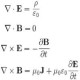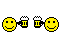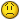NEW: Learning electronics? Ask your questions on the new Electronics Questions & Answers site hosted by CircuitLab.

Project Help and Ideas » Making a hydroponics plant grower

January 09, 2012
by TLRI have this idea of building a simple hydroponics system to grow small plants inside, at any time of the year.

Like strawberries in the winter time, or tomatos.

The system will use the atmega168 to control the amount of light, temperature control, watering, and ph levels. The LCD 20/4 screen will show the settings of ph, temperature,fan cooling, lighting on/off per days and also control amount of water needed per day.

Tom

January 09, 2012
by treymdI don't see any reason why it couldn't. You would of course need other components to drive things like the motors and lights, but the brains of the operation could be a 168.

January 09, 2012
by pcboltTom,

The ATmega168 has a multiplexed Analog-Digital converter with 6 usable input lines, so it should easily measure a pH sensor, a photocell, temp sensor (First Nerdkit project) and a water sensor (if needed). Timing would be easy as well, but if the power goes out, it would reset to 0, so you wouldn't have an absolute "clock". But that's a small matter. Sounds like a great project.

January 10, 2012
by TLRI have other programming skills, but this is my first project using C. I need a crash course to make this program. The microprocessor will have other drivers to control the light, pump, and cooling. The important part would the program, using a pre-program to adjust the settings while the system is running. So I would need a 4 key input to increase/decrease each state of the software. In othe words, if there is a arrow keys to move though the list using a up/down keys to search say for PH level, and using +/- to control ph level and so on. Each state would have a list like: Ph Level (1-9), cooling temp air (60f-100f), light on time (1hr-24hr), watering time (on/off),ground temp, and also clock to control time&day.

Tom

January 10, 2012
by TLRThis project will use very lower power no greater then 20watts, the size is 12"dia x 12" dome, and a 12" x 6" bass. I have a drawing, but don't know how to add it to my chat...

Cost is under \$50.00 Tom

January 10, 2012
by TLR<a href="http://imgur.com/Uu0UU"><img src="http://i.imgur.com/Uu0UU.jpg" alt="" title="Hosted by imgur.com" /></a>

January 10, 2012
by TLRhttp://i.imgur.com/Uu0UU.jpg

January 10, 2012
by TLRAlt Text

January 10, 2012
by RalphxyzSupported Markup

Images: ![alt image text](http://somepath.com/path/to/img.jpg) will include that image in your post.

See our How To on embedding images in a post.This is really neat. But don't you want a valve controlling the water not a switch?

Ralph

January 10, 2012
by TLRThanks Ralph, for posting my design. Yes, this one will use gravity feed to the plants, using a simple valve to open the watering port. I also have the same version without the gravity feed, using a small dc pump to feed water though the bottom too. The design is to make a hydroponics to grow inside plant any time of the year.

My goal is to grow strawberries in any home, at any time of the year...

Thanks again Ralph for help out on my post..

This is my engine project... http://rotarypistonengine.com/

Tommey Reed

January 10, 2012
by TLRJanuary 10, 2012
by TLRThis is a better design, using the arrows to move the list like" Temperature, lighting and so on. The -/+ will control the settings and the E will store it to that file.

The theory is to design a hydroponics system to control the growth of any plant, at any time of the year...

Tom

January 10, 2012
by RalphxyzI like your hydroponics system but that rotartypiston project is fascinating.

I want one.

Ralph

January 10, 2012
by TLRThank you..I needed a break that's why I'm building this project...

January 10, 2012
by TLRRalph, is there a software that will take a basic program and convert it to c for the atmega168?

I can program qbasic, bliz3d,and stamp2... Or will I have to do a lot of study on c?

Tom

January 10, 2012
by Rick_SBascomAVR is a basic compiler for the Atmel chips. You can use it to compile the hex file then use avrdude to upload it. If BASIC is what you want to program with, Bascom is the way to go. They have a free demo version of the compiler that isn't limited in any way other than the final compiled program size can be no larger than 4K.

Rick

January 10, 2012
by TLRMy 24hr clock for a timer

# include <LiquidCrystal.h>

int h1=0; int m1=0; int s1=0; int h2=1; int m2=0; int s2=0; int t1=0; int t2=0; int d=0;

// initialize the library with the numbers of the interface pins LiquidCrystal lcd(12, 11, 5, 4, 3, 2); void setup() { // set up the LCD's number of columns and rows: lcd.begin(16, 4); // Print a message to the LCD. lcd.setCursor(0, 0); lcd.print(">>>>> <<<<<"); }

void loop() { // set the cursor to column 0, line 1 // hour lcd.setCursor(5, 0); lcd.print(h1); lcd.setCursor(6, 0); lcd.print(h2); lcd.setCursor(7, 0); lcd.print(":"); //minute lcd.setCursor(8, 0); lcd.print(m1); lcd.setCursor(9, 0); lcd.print(m2); lcd.setCursor(10, 0); lcd.print(":"); //second lcd.setCursor(11, 0); lcd.print(s1); lcd.setCursor(12, 0); lcd.print(s2); if (d < 1) { lcd.setCursor(14,0); lcd.print("AM"); }

if (d > 0) { lcd.setCursor(15,0); lcd.print("PM"); }

//start timer s2=s2+1; delay(1000); //set second to count to o to 59 reset and add a minute; if (s2 > 9) { s2=0; s1=s1+1; } if (s1 > 5) { s1=0; m2=m2+1; }

if (m2 > 9) { m2=0; m1=m1+1; } if (m1 > 5) { m1=0; h2=h2+1; }

if (h2 > 9) { h2=0; h1=h1+1; } if (h1 > 0 and h2 > 2) { d=d+1; h1=0; m1=0; s1=0; h2=1; m2=0; s2=0; t1=0; t2=0; } //change d=0 (am) d=1(pm) if (d > 1) { d=0; } }

January 10, 2012
by TLRAlt Text

January 10, 2012
by TLRAlt Text

January 10, 2012
by TLRAlt Text

January 10, 2012
by TLRI tested this clock, to run 24hr and switching from am to pm...

I had to use different codes to control hr/min/sec= h1,h2:m1,m2:s1,s2 This is how I was able to tell the formula when 59 sec are up to reset and add 1 min.

This is the start on the microponics, I needed a timer to control input&output.

I will start adding the lighting control, temperature readings and so on..

tom

January 10, 2012
by pcboltHi Tom,

For someone learning C that's a pretty good start. I noticed you used the delay_ms() function in your code and while that works, your program won't be able to do anything else while that is being called. Since you'll be wanting to do many other things in that time, the best solution would be to use interrupts. See this Nerdkit tutorial.

Are you still going with the gravity feed? I've heard re-circulation is the key to good hydroponics.

January 11, 2012
by Rick_STom,

I know you are pretty new here so I hope you don't take this the wrong way. But when you post images and programs in the forum, PLEASE use the forum markup. Rather than seeing a linke to imgur, it's much better to seeAlso rather than the piled up blob of code like you have above, after you paste the code, select it and click the Indent Selection as Code Block button below. That will automatically indent each line so it makes the code readable.

You already know how to post a link, a photo is no different, you just need to add an exclamation point before the 1st bracket and use the TRUE url of the image.

Did you look into the BascomAVR program?? That was how I started because I was also much more versed in BASIC than C.

Rick

January 11, 2012
by Rick_SOn the subject of your project here. To do this well, you will want to become intimate with timers, and interrupts. You can have time based interrupts to perform functions at certain intervals, you can have pin change interrupts to perform functions when a certain event happens. Use your interrupts wisely. Don't do much in them, instead, set flags that allow the main program to branch to a function after the interrupt has occurred. The main reason for this is that while in an interrupt, all other interrupts are suspended. So Interrupt code should be kept as small as possible to allow the program to continue it's course.

Rick

January 11, 2012
by TLR# include <LiquidCrystal.h>

int h1=0; int m1=0; int s1=0; int h2=1; int m2=0; int s2=0; int t1=0; int t2=0; int d=0;

// initialize the library with the numbers of the interface pins LiquidCrystal lcd(12, 11, 5, 4, 3, 2); void setup() { // set up the LCD's number of columns and rows: lcd.begin(16, 4); // Print a message to the LCD. lcd.setCursor(0, 0); lcd.print(">>>>> <<<<<"); }

void loop() { // set the cursor to column 0, line 1 // hour lcd.setCursor(5, 0); lcd.print(h1); lcd.setCursor(6, 0); lcd.print(h2); lcd.setCursor(7, 0); lcd.print(":"); //minute lcd.setCursor(8, 0); lcd.print(m1); lcd.setCursor(9, 0); lcd.print(m2); lcd.setCursor(10, 0); lcd.print(":"); //second lcd.setCursor(11, 0); lcd.print(s1); lcd.setCursor(12, 0); lcd.print(s2); if (d < 1) { lcd.setCursor(14,0); lcd.print("AM"); }

if (d > 0) { lcd.setCursor(15,0); lcd.print("PM"); }

//start timer s2=s2+1; delay(1000); //set second to count to o to 59 reset and add a minute; if (s2 > 9) { s2=0; s1=s1+1; } if (s1 > 5) { s1=0; m2=m2+1; }

if (m2 > 9) { m2=0; m1=m1+1; } if (m1 > 5) { m1=0; h2=h2+1; }

if (h2 > 9) { h2=0; h1=h1+1; } if (h1 > 0 and h2 > 2) { d=d+1; h1=0; m1=0; s1=0; h2=1; m2=0; s2=0; t1=0; t2=0; } //change d=0 (am) d=1(pm) if (d > 1) { d=0; } }

January 11, 2012
by TLRHow do you remove unwanted post? I made a mistake again...

January 11, 2012
by TLRYes, I'm going to use re-circulation in the hydroponics.

January 11, 2012
by Rick_STLR said:

"How do you remove unwanted post? I made a mistake again..."

1st off, don't worry about it, sometimes those of us who've been here a while may get grumpy, but overall, we try to be friendly.I just suggested what I did earlier to make it easier for people who might want to jump in and help you, have an easier time of it. I'm sure you've seen code posted in other threads as code and can see the difference. Without formatting it as code, it just becomes a jumbled mess and very hard to follow. It really isn't that difficult once you get the hang of it.

To format the text as code, you simply paste the code you want to display into the post. Then select all the code by clicking at the beginning of the code and dragging to the end of the code. (this will highlight it) Then press the Indent Selection as Code Block button below the Preview and Post buttons. This should shift the code over.

Once that is finished, press the Preview button and look at the results. If it isn't formatted properly in the preview screen, it won't be in the final post. So before hitting the post button, make any changes needed to get it looking the way you want.

As for removing or editing unwanted posts, you can't unfortunately.This forum doesn't have edit abilities once you hit the post button. That is something all of us who've been here a while have longed for.

Rick

January 11, 2012
by Rick_SAlso, looking at your code above, are you programming in arduino? I noticed liquidcrystal.h at the top of your file and a setup function. Is there a reason you aren't using native C?

January 11, 2012
by TLRI'm working with all three microprocessor. nerdkit,arduino and stamp are a great way to testing programming skils, and also a way to learn different styles of code. So yes, that code was a arduino version, but I'm also working on native C version.

January 11, 2012
by TLR``````#include <LiquidCrystal.h>
int h1=0;
int m1=0;
int s1=0;
int h2=1;
int m2=0;
int s2=0;
int t1=0;
int t2=0;
int d=0;
int q1=10;
int q2=1;
const int pin1 = 7;
int pin1state = 0;

const int pin2 = 8;
int pin2state = 0;

// initialize the library with the numbers of the interface pins
LiquidCrystal lcd(12, 11, 5, 4, 3, 2);
void setup() {
// set up the LCD's number of columns and rows:
lcd.begin(16, 4);
// Print a message to the LCD.
lcd.setCursor(0, 0);
lcd.print(">>>>>          <<<<<");
//input keys

pinMode (pin1,INPUT);
}

void loop() {
//up button to cycle list upward
if (pin1state == HIGH){
q1=q1+1;
}
if (q1>20)
{
q2=q2+1;
q1=10;
}

//down button to cycle list downward
if (pin2state == HIGH){
q1=q1-1;
}
if (q1<0)
{
q2=q2-1;
q1=10;
}
//sets cycle limit to 5 types of lists
if (q2 <1)
{
q2=5;
}
if (q2 >5)
{
q2=1;
}
//list each cycle and prints on lcd q2=1 to q2=5
if (q2==1)
{
lcd.setCursor(0, 2);
lcd.print(" Ground Temperature ");
lcd.print("      70 deg F      ");
}
//q2=2
if (q2==2)
{
lcd.setCursor(0, 2);
lcd.print("  Air Temperature   ");
lcd.print("     72 deg F       ");
}
if (q2==3)
{
lcd.setCursor(0, 2);
lcd.print("   Water PH Level   ");
lcd.print("      5.5-6.0       ");
}
if (q2==4)
{
lcd.setCursor(0, 2);
lcd.print(" Water on/off Timer ");
lcd.print("    20 second       ");
}
if (q2==5)
{
lcd.setCursor(0, 2);
lcd.print(" Light on/off Timer ");
lcd.print("   9hr/per/day      ");
}

// set the cursor to column 0, line 1
// hour
lcd.setCursor(5, 0);
lcd.print(h1);
lcd.setCursor(6, 0);
lcd.print(h2);
lcd.setCursor(7, 0);
lcd.print(":");
//minute
lcd.setCursor(8, 0);
lcd.print(m1);
lcd.setCursor(9, 0);
lcd.print(m2);
lcd.setCursor(10, 0);
lcd.print(":");
//second
lcd.setCursor(11, 0);
lcd.print(s1);
lcd.setCursor(12, 0);
lcd.print(s2);
if (d < 1)
{
lcd.setCursor(14,0);
lcd.print("AM");
}

if (d > 0)
{
lcd.setCursor(15,0);
lcd.print("PM");
}

//start timer
t1 = t1 + 1;
if (t1 > 75)
{
s2 = s2 +1;
t1 = 0;
}

//set second to count to o to 59 reset and add a minute;
if (s2 > 9)
{
s2=0;
s1=s1+1;
}
if (s1 > 5)
{
s1=0;
m2=m2+1;
}

if (m2 > 9)
{
m2=0;
m1=m1+1;
}
if (m1 > 5)
{
m1=0;
h2=h2+1;
}

if (h2 > 9)
{
h2=0;
h1=h1+1;
}
if (h1 > 0 and h2 > 2)
{
d=d+1;
h1=0;
m1=0;
s1=0;
h2=1;
m2=0;
s2=0;
t1=0;
t2=0;
}
//change d=0 (am) d=1(pm)
if (d > 1)
{
d=0;
}
}
``````